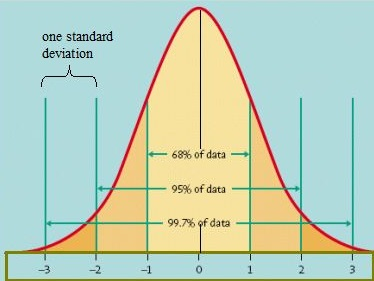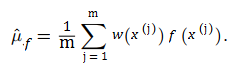# Importance Sampling: Simple Definition

Importance sampling is a way to predict the probability of a rare event. Along with Markov Chain Monte Carlo, it is the primary simulation tool for generating models of hard-to-define probability distributions.Just 3 in 1000 events are found in the extreme tails on a bell curve (±3 Standard Deviations). Image credit: University of Virginia.

Rare events can usually be found on the tails of probability distributions. For example, on a bell curve for IQ, the Albert Einsteins of the world are found above three standard deviations from the mean. The rarity of finding results like this makes it extremely difficult to sample large enough numbers for any meaningful statistical analysis. In addition, the probability distribution for these rare events are going to look markedly different from the bell curve. Although predicting when another Einstein might be born is probably not that critical, predicting other rare events — like fatigue in engineering structures or landfall for category 5 hurricanes — can be a matter of life and death.

As well as finding probabilities in tails, importance sampling can also be used to find expectations of random functions.

## Biasing Density

One way to produce large enough samples is to change the probability density function to generate more rare events. This alternate density function is derived from the original function of interest (in the above example, the bell curve) and is usually called the biasing density. The end goal is to reduce the variance of your estimates. The basic steps are:

1. Choose a model for the process you want to study (i.e. derive the biasing density function and define the model’s parameters (e.g. the mean and variance)),
2. Draw random samples from the parameterized model,
3. Run your statistical analysis on the biasing density function,
4. Modify those results to reflect the changes you made to the probability distribution.
5. Analyze the output.

## Importance Sampling and Monte Carlo Procedures

Importance sampling speeds up Monte Carlo procedures for rare events (a “Monte Carlo procedure” is sampling based on random walks). As it speeds up the process, it’s sometimes referred to as “fast simulation using importance sampling.” It’s also called a “forced Monte Carlo procedure” because it’s forcing the Monte Carlo procedure to behave somewhat abnormally.

If you’re using Monte Carlo procedures, you’re more than likely using software because of the large number of computations involved. Many statistical software packages include Monte Carlo algorithms, including Minitab, R and SPSS.

## Formulas

The formulas behind Importance Sampling are somewhat esoteric, mainly because of the calculus involved. As a (relatively) simple example, let’s say you wanted to create an expectation for some function, f:
μf = ℰp[f(X)], with

μf = ∫ f(x)p(x)dx

Then for any probability density function q(x) that satisfies q(x) > 0 when f(x)p(x)≠ 0, you have:

μf = ℰq[w(X)f(X)]

Where:

• w(x) = p(x)/q(x)
• q[] = expectation with respect to q(x).

You can now use a sample of independent draws from q(x) to estimate μf byReferences:
Neal, R. M. (2001). Annealed Importance Sampling. Statistics and
Computing (11) 125-139.
Oh, M.-S. and Berger, J. O. (1992). Adaptive Importance Samplin in
Monte Carlo Integration. Journal of Statistical Computation and Simulation
(41) 143-168.
Srinivasan, R. (2013). Importance Sampling: Applications in Communications and Detection. Springer Science & Business Media.
Tokdar, S. & Kass, R. (2009). Importance Sampling: A Review. Retrieved 8/18/2017 from: http://www2.stat.duke.edu/~st118/Publication/impsamp.pdf

CITE THIS AS:
Stephanie Glen. "Importance Sampling: Simple Definition" From StatisticsHowTo.com: Elementary Statistics for the rest of us! https://www.statisticshowto.com/importance-sampling/